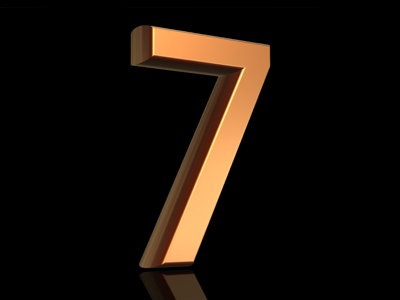3 x 213 = 7

# Multiples 3 (Easy)

This is the third in our Easy section of Eleven Plus maths quizzes on Multiples. In it we shall ask you to find common multiples, and odd men out, as well as giving you some tips to help you in your exams. We’ll also be looking at multiples of fractions and decimal numbers.

That’s right – fractions and decimals do have multiples, which seems odd given that whole numbers cannot have fractions as their multiples. It’s all to do with whether or not a number can be divided by another without leaving a remainder. So, 212 is a multiple of 12 because 5 x 12 = 212. However, 212 is NOT a multiple of 5. That’s because 5 x 0.5 = 212 and 0.5 is not a whole number.

Okay, now we’ve cleared that up, it’s time for the quiz. Read all the questions carefully – there are some crafty onesto fool the careless. Don't let them fool you!

1.
A number is a multiple of 2 if …?
It is even
Its last two digits are divisible by 2
It ends with an 8
All of the above
The quickest way to work out whether or not a number is a multiple of two is to ask yourself, “Is the number even?”
2.
Which of the four choices is NOT a multiple of ALL the numbers given?
4, 7, 6
42
84
168
252
42 is not divisible by 4. Sometimes the times tables don’t go up high enough – if that is the case then you’ll have to do some calculations. Just divide th large numbers by 4, 7 and 6 to find out whether they are multiples
3.
Which number is a multiple of 213?
6
7
8
9
3 x 213 = 7
4.
Which number is a multiple of 17?
52
69
86
102
6 x 17 = 102. None of the other options appears in the 17 times table
5.
A number is a multiple of 8 if …?
The last three digits are divisible by 8
The last two digits are divisible by 8
The last digit is divisible by 8
All of the above
That’s because 8 goes into 200 but not into 100: 624 ÷ 8 = 78 BUT 524 ÷ 8 = 65.5
6.
Which of the four choices is a multiple of ALL the numbers given?
1.2, 5, 12
6
12
24
60
6 is not divisible by 12 or 5, while 12 and 24 are not divisible by 5
7.
Which of the four choices is a multiple of ALL the numbers given?
2.5, 4, 5
10
15
25
35
15, 25 and 35 are not divisible by 4
8.
Which of the four choices is NOT a multiple of ALL the numbers given?
20, 30, 60
60
120
140
180
140 is not divisible by 60 or 30, just like 14 is not divisible by 6 or 3. If all three numbers end with a 0 you can ignore them
9.
Which list is made up of multiples of 16?
64, 68, 72, 76
80, 88, 96, 104
72, 84, 96, 108
80, 96, 112, 128
The other three options are taken from the 4, 8 and 12 times tables
10.
Which list is made up of multiples of 4?
8, 28, 34, 72
16, 40, 48, 78
20, 36, 52, 84
24, 42, 56, 80
34, 42 and 78 cannot be divided by 4 without a remainder
Author:  Frank Evans

We use cookies to make your experience of our website better.

To comply with the new e-Privacy directive, we need to ask for your consent -